# TS 10th Class Physical Science Important Questions Chapter 2 Chemical Equations

These TS 10th Class Physical Science Chapter Wise Important Questions Chapter 2 Chemical Equations will help the students to improve their time and approach.

## TS 10th Class Physical Science Important Questions Chapter 2 Chemical Equations

1 Mark Questions

Question 1.
What are the reactants and products in a chemical reaction.
The substances which undergo chemical changes In a reaction are called reactants and the new substances formed are called products.

Question 2.
What Is meant by balanced equation?
A chemical equation Is said to be balanced when the number of atoms of each element is same on both reactant side and product side.

Question 3.
If you keep an iron piece in solid-state CuSO4 crystals, does it get any reaction? Guess the reason.
Reaction will not takes place if an ¡ron piece is placed in solid state CuSO4 crystals because there will not exist separate Cu2+, SO4-2 ions in CuSO4 crystals. In aqueous solution, they exists. So iron cannot displace copper.

Question 4.
Baiancethefollowlng equations.
(1) Na + O2 → Na2O
(2) H2O2 → H2O + O2
(3) Mg(OH)2 + HCl → MgCl2 + H2O
(4) Fe + O2 → Fe2O3
(1) 4Na + O2 → 2Na2O
(2) 2H2O2 → 2H2O + O2
(3) Mg(OH)2+ 2HCl → MgCl2 + 2H2O
(4) 4Fe + 3O2 → 2Fe2O3Question 5.
Balance the following equations. (AS1)
(1) Al(OH)3 → Al2O3 + H2O
(2) NH3 + CuO → Cu + N2 + H2O
(3) Al2(SO4)3 + NaOH → Al(OH)3 + Na2SO4
(4) HNO3 + Ca(OH)2 → Ca(NO3)2 + H2O
(5) NaOH + H2SO4 → Na2SO4 + H2O
(6) BaCl2 + H2SO4 → BaSO4 + HCl
(1) 2Al(OH)3 → Al2O3 + 3H2O
(2) 2NH3 + 3CuO → 3Cu + N2 + 3H2O
(3) Al2(SO4)3+ 6NaOH → 2Al(OH)3 + 3Na2SO4
(4) 2HNO3 + Ca(OH)2 → Ca(NO3)2 + 2H2O
(5) 2NaOH + H2SO4 → Na2SO4 + 2H2O
(6) BaCl2 + H2SO4 → BaSO4 + 2HCl

Question 6.
What is a chemical equation?
Describing a chemical reaction using least possible words or symbols is called a chemical equation.
Ex : CaO + H2O → Ca(OH)2

Question 7.
MnO2 + 4HCl → MnCl2+ 2H2O + Cl2. In the given equation, name the compound which is oxidized and which is reduced?
In the above equation Ha compound is oxidized and MnO2 is reduced.

Question 8.
What happens If the copper sulphate crystals taken into dry test tube are heated?

1. When copper sulphate crystals are heated, water present in crystals is evaporated and the salt turns white.
2. Evaporated water appears as droplets on the walls of the test tube.
3. Blue-coloured copper sulphate (CuSO45H2O) is turned into white colour beacuse 5H2O molecules are evaporated from crystals.

Question 9.
Write the equation for the chemical decomposition reaction of silver chloride In the presence of sunlight.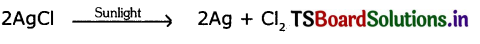2 Marks Questions

Question 1.
What do you observe during chemical change?
During chemical change, we observe that

1. The original substances have their characteristic properties. Hence these may be products with different physical states colours and different compositions.
2. Chemical changes may be exothermic or endothermic.
3. They may form an Insoluble substance known as precipitate.
4. There may be gas liberation in a chemical danger.

Question 2.
How do you Write a chemical equation?

1. A chemical reaction, written tri the form of formula equation, shows the change of reactants to products by an arrow placed between them.
2. The reactants are written on the left side of arrow and the final substances or products are written on the right side of the arrow.
3. The arrowhead points towards the products and shows the direction of reaction.
4. If there are more than one reactant or product involved in the reaction, they are separated with a plus (+) sign between them.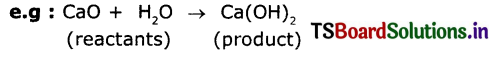Question 3.
What are the effects of oxidation on everyday life?
1. Combustion is the most common example for oxidation reactions.
2. Rising of dough with yeast depends on oxidation of sugars to carbon dioxide and water.
3. Metals are corroded due to oxidation.
4. Bleaching of coloured objects using moist chlorine.
Cl + H2O → HOCl + HCl
HOCl → HCl + (O)
Coloured object + (0) → Colourless object (Here O’ is known as Nascent oxygen)
5. Sometimes during rainy season the power supply to our homes from the electric pole will be interrupted due to formation of metal oxide layer on the electric wire. This metal oxide is an electrical insulator. On removing the metal oxide layer formed on the wire with sandpaper, supply of electricity can be restored.Question 4.
What are the important characteristics of chemical reactions?
The important characteristics of chemical reactions are

1. Evolution of a gas
2. Formation of a precipitate
3. Formation of new substances,
4. Change in colour
5. Change in temperature
6. Change in state

Question 5.
Write some chemical reactions occurring in our daily life.

1. Souring of milk
2. Formation of curd from milk
3. Cooking of food
4. Digestion of food in our body
5. Fermentation of grapes
6. Rusting of iron
7. Burning of fuels
8. Burning of cooking gas
9. Ripening of fruits.

Question 6.
What symbols do we use to indicate the physical state of reactants and products In an equation?

1. Solid state is indicated by the symbol (s)
2. Liquid state is indicated by the symbol ( l )
3. Gaseous state is indicated by the symbol (g)
4. Aqueous solution is indicated by the symbol (aq)
5. Precipitate Is Indicated by an arrow with head downwards (↓)

Question 7.
Comment on “C(s) + O2(g) → CO2(g) + Heat’ equation.

1. The burning of carbon In oxygen Is an exothermic reaction because heat is evolved in this reaction.
2. An exothermic reaction is indicated by writing + Heat or + Heat energy or just+ Energy on product side of an equation.

Question 8.
Comment on ‘N2(g) + O2(g) + Heat → 2NO(g)” equation.

1. The reaction between nitrogen and oxygen to form Nitrogen Monoxide is endothermic reaction because heat is absorbed n this reaction.
2. An endothermic reaction is usually indicated by writing + Heat or Just ‘+ Energy on the reactants side of an equation.

Question 9.
2Cu + O2 → 2CuO What information do you get from above equation?
The above equation tells us that.

1. Copper reacts with oxygen to form copper oxide.
2. The formula of copper oxide.is, CuO and that of oxygen is
3. 2 moles of copper atoms react with 1 mole of oxygen molecules (O2) to produce 2 moles of copper oxide (CuO).

Question 10.
Balance the following chemical equation and follow the steps involved in balancing a chemical equation.
Cu2S + O2 → Cu2O + SO2
Step 1: Write the unbalanced equation using correct chemical formula for all substances
Cu2S + O2 → Cu2O + SO2
Step 2: Compare no. of atoms of each element on both sides.

 Atom No. of atoms in L.H.S. No. of atoms in R.H.S. Cu 2 2 S 1 1 O 2 3

Balancing Cu, S, O atoms both sides
2Cu2S + 3O2 → 2Cu2O + 2SO2
The equation is balanced.

Step 3: Write the co-efficient smallest ratio.
2Cu2S +3O2 → 2Cu2O + 2SO2

Step 4: Verify above equation for balancing of atom of each element on both sides.
2Cu2S + 3O2 → 2Cu2O + 2SO2

Question 11.
Observe the following balanced chemical equation and answer the given questions.
C3H8(8) + 5O2(g) → 3CO2(g) + 4H2O(g)
(i) How many molecules of Oxygen are involved In this chemical reaction?
(ii) How many moles of Propane are required to get 20 moles of Water?
(i) In this chemical reactIon five molecules of oxygen are involved.
(ii) Five moles of propane are required to get 20 moles of water.

Question 12.
Write the products of given reactions, if any. Give reason.
FeCl2 + Zn →
ZnCl2 + Fe →
FeCl2 + Zn → ZnCl2+Fe (Displacement reaction)
ZnCl2 + Fe → No reaction. (Low reactive metals cannot displace high reactive metals).Question 13.
Balance the following chemical equations:
(i) Na+ H2O → NaOH+H2
(ii) K2CO3+HCl →KCl+H2O+CO2
(i) 2Na + 2H2O → 2NaOH + H2
(ii) K2CO3 +2HCl → 2KCl + H2O+CO2

4 Marks Questions

Question 1.
What are the materials required for the experiment to show the chemical decomposition of water? Write the procedure of the experiment. Name the products which we get in this reaction.
Material required for chemical decomposition of water:
A plastic mug, two carbon rods, two corks, two test tubes, connecting wires, 9V battery, water, and some drops of acid.

Procedure:

1. Take a plastic mug, drill holes at the base.
2. Fit two one-holed rubber stoppers in these holes.
3. Insert two carbon electrodes in these rubber stoppers.
4. Connect the electrodes to 9V battery.
5. Fill the mug with water, so that the electrodes are immersed.
6. Add few mops of any acid.
7. Take two test tubes tilled with water and insert them over the two carbon electrodes.
8. Switch or’ the circuit and leave the apparatus undisturbed for some time.
9. We notice the liberation of bubbles at both the electrodes. These bubbles displace the water in the test tubes.
10. After the test tubes are filled with gas, take them out and test the gases with the burning match Stick.

The products which we get in this reaction:
The gases are condensed oxygen and hydrogen.

Question 2.
Explain the steps involved in balancing a chemical equation with an example.
(or)
Why should we balance a chemical equation? Take any one chemical equation and explain the procedure of balancing it.
A chemical equation in which the number of atoms of different elements on the reactants side are same as those on product side is called a balanced equation.
Steps involved In balancing a chemical reaction: Let us consider the combustion reaction of Propane.
Step 1: Write the unbalanced equation using correct chemical formulae for all substances.
C3H8 + O2 → CO2 + H2O (Skeleton equation)

Step 2: Compare number of atoms of each element on both sides.Find the coefficients to balance the equation. In this case, there are 3 carbon atoms on the left side of the equation but only one on the right side. If we add a coefficient of 3 to CO2 on the right side the carbon atoms balance.
C3H8 + O2 → 3CO2 + H2O
Now, look at the number of hydrogen atoms. There are 8 hydrogen atoms on the left but only 2 on the right side. By adding a coefficient of 4 to the H2O on the right side, the hydrogen atoms get balanced.
C3H8+ O2 → 3CO2 + 4H2O
Finally, look at the number of oxygen atoms. There are 2 on the left side but 10 on the right side. By adding a coefficient of 5 to the 02 on the left side, the oxygen atoms get balanced.
C3H8 + 5O2 → 3CO2 + 4H2O

Step 3: Make sure the coefficients are reduced to their smallest whole number values. The above equation is already with the coefficients in smallest whole numbers. There is no need to reduce its coefficients. Hence the final equation is
C3H8 + 5O2 → 3CO2 + 4H2O

Step 4: Check the answer. Count the numbers and kinds of atoms on both sides of the equation to make sure they are the same.

Question 3.
Chemical equations can be made more informative by expressing the following characteristics of the reactants and products.
(i) Physical state
(ii) Heat exchange (exothermic or endothermic change)
(iii) Gas evolved (if any)
(iv) Precipitate formed (If any)
(i) Expressing the physical state: The physical state of the substances maybe mentioned along with their chemical formulae. The different states i e., gaseous, liquid and solid states are represented by the notations(g), (I) and (s) respectively. If the substance is present as a solution in water, it Is represented as (aq).
Eg : Fe2O3(s) + 2Al(s) → 2Fe(s) +Al2O< sub>3(s)

(ii) Heat exchange: Heat is liberated in exothermic reactions and heat is absorbed in endothermic reactions.
Eg: 1. C(s) + O2(g) → CO2(g) + Q (exothermic reaction)
2. N2(g)+ O2(g) → 2NO(g) — Q (endothermic reaction)
(or)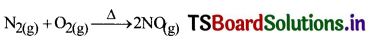(iii) Gas evolved: If a gas is evolved in a reaction, it is denoted by an upward arrow ↑or (g)
Eg: Zn(s) + H2SO4(aq) → ZnSO4(aq) + H2(g)

(iv) Precipitate formed: If a precipitate is formed in the reaction, it Is denoted by a downward arrow.
Eg : AgNO3(aq) + NaCl(aq) → AgCl(s) ↓ + NaNO3(aq)

Question 4.
Balance the following chemical equations.
(a) Na2SO4 + BaCl2 → BaSO4 + NaCl
(b) Al4C3 + H2O → CH4 + Al(OH)3
(c) Pb(NO3)2 → PbO + NO2 + O2
(d) Fe2O3 + Al → Al2O3 + Fe
(a) Na2SO4 + BaCl2 → BaSO4 + 2NaCl
(b) Al4C3 + 12H2O → CH4 + 4Al(OH)3
(c) 2Pb(NO3)2 → 2PbO + 4NO2 + O2
(d) Fe2O3 + 2Al → Al2O3 + 2FeSolved Problems

Question 1.
Al(s) + Fe2O3(s) → Al2O3(s) + Fe(s) (atomic masses of Al = 27U, Fe = 56U, and O = 16U)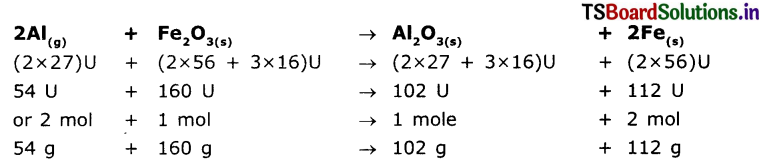Suppose that you are asked to calculate the amount of aluminum, required to get 1120 kg of iron by the above reaction.
Solution:
As per the balanced equation
Aluminium → Iron
54g → 112g
X? → (1120 x 1000)g
∴ xg = $$\frac{(1120 \times 1000) \mathrm{g} \times 54}{112 \mathrm{~g}}$$ = 10000 x 54g = 540000 g (or) 540 kg.
To get 1120 kg of iron we have to use 540 kg of aluminum.

Question 2.
Calculate the volume, mass, and number of molecules of hydrogen liberated when 230 g of sodium reacts with excess of water at STP. (atomic masses of Na = 23U), O=16U, and H=1U)
The balanced equation for the above reaction is,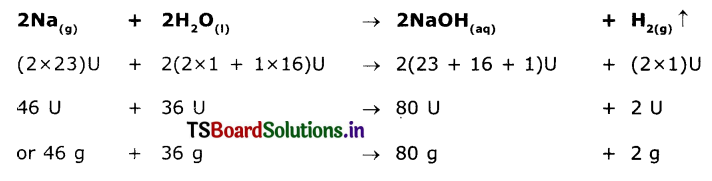Solution:
As per the balanced equation
46g of Na gives 2g of hydro9en.
230g of Na gives …………………? g of hydrogen.
$$\frac{230 \mathrm{~g} \times 2 \mathrm{~g}}{46 \mathrm{~g}}$$ = 10g of hydrogen.
I gram molar mass of any gas at STP ie, standard temperature 273K and standard pressure 1 bar occupies 22.4 liters known as gram molar volume.

2.0 g of hydrogen occupies 22.4 liters at STP.
10.0 g of hydrogen occupies…… ? litres at STP.
$$\frac{10.0 \mathrm{~g} \times 22.4 \text { litres }}{2.0 \mathrm{~g}}$$ = 112 litres
2 g of hydrogen i.e, 1 mole of H2 contains 6.02 x 1023 (H2) molecules 10 g of hydrogen contain ……………………. ?
$$\frac{10.0 \mathrm{~g} \times 6.02 \times 10^{23} \text { molecules }}{2.0 \mathrm{~g}}$$ = 30.10 x 1023 molecules = 3.01 × 1024 molecules

Question 3.
Calculate the volume and No. of molecules of CO2 liberated at STP if 50g of CaCO3 is treated with dilute hydrochloric acid which contains 7.3 g of dissolved HCI gas.
Solution:
The Chemical equation for the above the relation is
CaCO3(s) + 2HCl (aq) → CaCl2(aq) + H2O(l) + CO2(g)
As per the stoichiometric equation, 100 g of CaCO3 reacts with 73 kg of HCl to liberate 44 g of CO2.
In the above problem the amount of CaCO3, taken is 50 gm and HCl available is 7.3 g
100g of CaCo3, require 73g of HCl and 50 g of CaCo3 required 36.6 g of HCl but, only 7.3 g of HCI is available.
Hence the product CO2 formed depends only on the amount of HCl which Is in the the least amount but not on the amount of CaCO3 which is an excess. The reactant available in less amount Is called limiting reagent as it limits the amount of prouct formed.

Therefore, we can write
73 g of HCl → 44 g CO2
7.3g of HCl- ?
$$\frac{7.3 g \times 44 g}{73 g}$$ = 4.4 g
44 g of CO2 occupies 22.4 L volume at STP
4.4 g of CO2 occupies – ?
$$\frac{4.4 g \times 22.4 L}{44 g}$$ = 2,24L
44 g of CO2 contain 6.023 × 1023 mol of CO2 4.4 g Contains – ?
$$\frac{4.4 g \times 6.023 \times 10^{23}}{44 g}$$ = 6.023 × 1022 molQuestion 4.
Write the equation for the reaction of Zinc with hydrochloric acid and balance the equation. Find out the number of molecules of hydrogen gas produced In this reaction, when 1 mole of HCl completely reacts at S.T.P. [Gram molar volume is 22.4 liters at S.T.P., Avogadro’s number is 6.023 x1023]
1 moIe of HCl produces $$\frac{1}{2}$$ mole of H2 gas
$$\frac{1}{2}$$ mole of H2 gas contains = $$\frac{6.023 \times 10^{23}}{2}$$